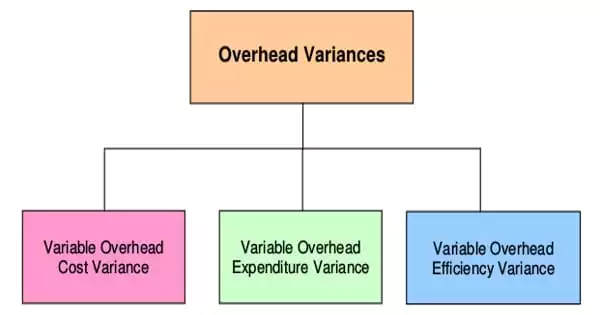AccountingThe difference between actual and applied overhead is referred to as overhead variance. The overhead variance is the difference between the standard overhead costs allowed for the actual output achieved and the actual overhead costs incurred. In budgeting or management accounting, variance analysis is the study of deviations between actual and forecasted or planned behavior. Overhead variance can only be calculated after you have determined the actual overhead costs for the period. This is primarily concerned with how the difference between actual and planned behaviors affects business performance.

Overhead is calculated using a fixed rate and a cost driver. This is essentially a method of estimating overhead costs prior to incurring them. At the end of the fiscal period, the actual overhead costs can be compared to the predetermined estimates. The overhead variance is the difference between the actual overhead costs and the applied overhead costs. It is defined as the sum of indirect material, labor, and expense costs. Overhead variances may occur as a result of a discrepancy between the standard overhead costs budgeted and the actual overheads incurred.

The difference between the standard cost of overhead allowed for the actual output achieved and the actual overhead cost incurred is defined as overhead cost variance. In other words, overhead cost variance is the difference between under and over-absorption of overheads. Overhead variances can be classified as follows in order to convey useful information for controlling overhead expenses:

• Capacity or Volume variance,
• Spending variance,
• Efficiency variance.

Overhead cost variance can be classified as:

• Variable Overhead Variance: The difference between the standard variable overhead cost and the actual variable overhead cost. Because variable overhead costs vary in proportion to production, only a change in expenditure can cause such variance.
• Fixed Overhead Variance: The portion of total overhead cost variance resulting from the difference between the standard cost of fixed overhead allowed for the actual output achieved and the actual fixed overhead cost incurred.

Companies prepare a flexible budget that includes estimated revenues and costs at various levels of production to determine the overhead standard cost. The standard overhead cost is usually expressed as the sum of the fixed and variable costs per unit. Because total fixed costs are the same at different levels of production, the standard fixed cost per unit will vary depending on the level of production. The variable standard cost per unit, on the other hand, is the same for each level of production, but the total variable costs will vary.